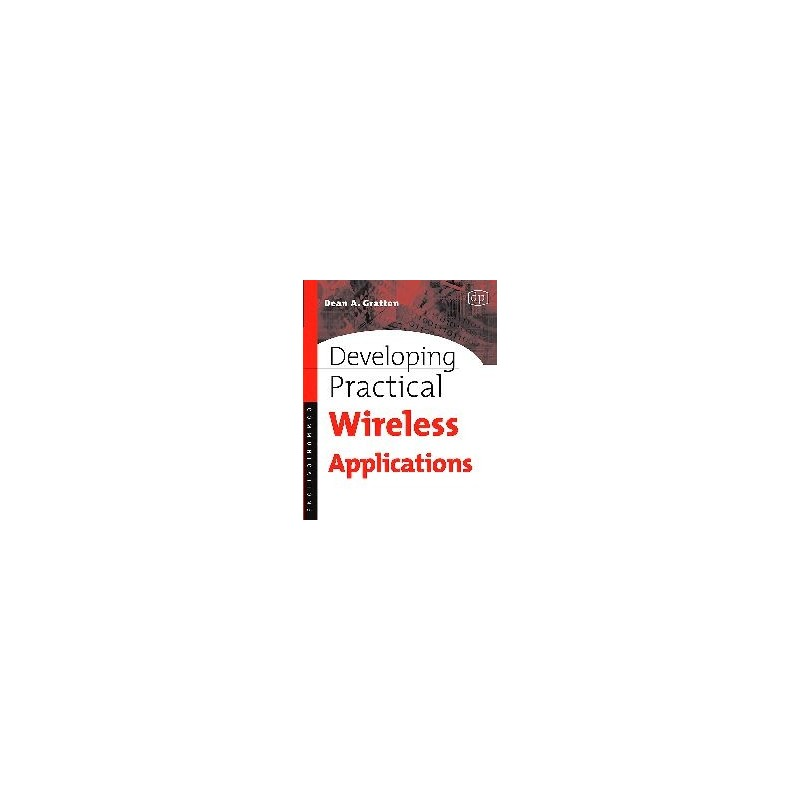﻿
Obniżka!Zobacz większe

# Residuated Lattices: An Algebraic Glimpse at Substructural Logics

Nikolaos Galatos, Peter Jipsen, Tomasz Kowalski, Hiroakira Ono

ID: 174732

### Więcej informacji

The book is meant to serve two purposes. The first and more obvious one is to present state of the art results in algebraic research into residuated structures related to substructural logics. The second, less obvious but equally important, is to provide a reasonably gentle introduction to algebraic logic. At the beginning, the second objective is predominant. Thus, in the first few chapters the reader will find a primer of universal algebra for logicians, a crash course in nonclassical logics for algebraists, an introduction to residuated structures, an outline of Gentzen-style calculi as well as some titbits of proof theory - the celebrated Hauptsatz, or cut elimination theorem, among them. These lead naturally to a discussion of interconnections between logic and algebra, where we try to demonstrate how they form two sides of the same coin. We envisage that the initial chapters could be used as a textbook for a graduate course, perhaps entitled Algebra and Substructural Logics.
As the book progresses the first objective gains predominance over the second. Although the precise point of equilibrium would be difficult to specify, it is safe to say that we enter the technical part with the discussion of various completions of residuated structures. These include Dedekind-McNeille completions and canonical extensions. Completions are used later in investigating several finiteness properties such as the finite model property, generation of varieties by their finite members, and finite embeddability. The algebraic analysis of cut elimination that follows, also takes recourse to completions. Decidability of logics, equational and quasi-equational theories comes next, where we show how proof theoretical methods like cut elimination are preferable for small logics/theories, but semantic tools like Rabin's theorem work better for big ones. Then we turn to Glivenko's theorem, which says that a formula is an intuitionistic tautology if and only if its double negation is a classical one. We generalise it to the substructural setting, identifying for each substructural logic its Glivenko equivalence class with smallest and largest element. This is also where we begin investigating lattices of logics and varieties, rather than particular examples. We continue in this vein by presenting a number of results concerning minimal varieties/maximal logics. A typical theorem there says that for some given well-known variety its subvariety lattice has precisely such-and-such number of minimal members (where values for such-and-such include, but are not limited to, continuum, countably many and two). In the last two chapters we focus on the lattice of varieties corresponding to logics without contraction. In one we prove a negative result: that there are no nontrivial splittings in that variety. In the other, we prove a positive one: that semisimple varieties coincide with discriminator ones.

Within the second, more technical part of the book another transition process may be traced. Namely, we begin with logically inclined technicalities and end with algebraically inclined ones. Here, perhaps, algebraic rendering of Glivenko theorems marks the equilibrium point, at least in the sense that finiteness properties, decidability and Glivenko theorems are of clear interest to logicians, whereas semisimplicity and discriminator varieties are universal algebra par exellence. It is for the reader to judge whether we succeeded in weaving these threads into a seamless fabric.

- Considers both the algebraic and logical perspective within a common framework.
- Written by experts in the area.
- Easily accessible to graduate students and researchers from other fields.
- Results summarized in tables and diagrams to provide an overview of the area.
- Useful as a textbook for a course in algebraic logic, with exercises and suggested research directions.
- Provides a concise introduction to the subject and leads directly to research topics.
- The ideas from algebra and logic are developed hand-in-hand and the connections are shown in every level.

Contents

List of Figures

List of Tables

Introduction

Chapter 1. Getting started

Chapter 2. Substructural logics and residuated lattices

Chapter 3. Residuation and structure theory

Chapter 4. Decidability

Chapter 5. Logical and algebraic properties

Chapter 6. completions and finite embeddability

Chapter 7. Algebraic aspects of cut elimination

Chapter 8. Glivenko theorems

Chapter 9. Lattices of logics and varieties

Chapter 10. Splittings

Chapter 11. Semisimplicity

Bibliography

Index